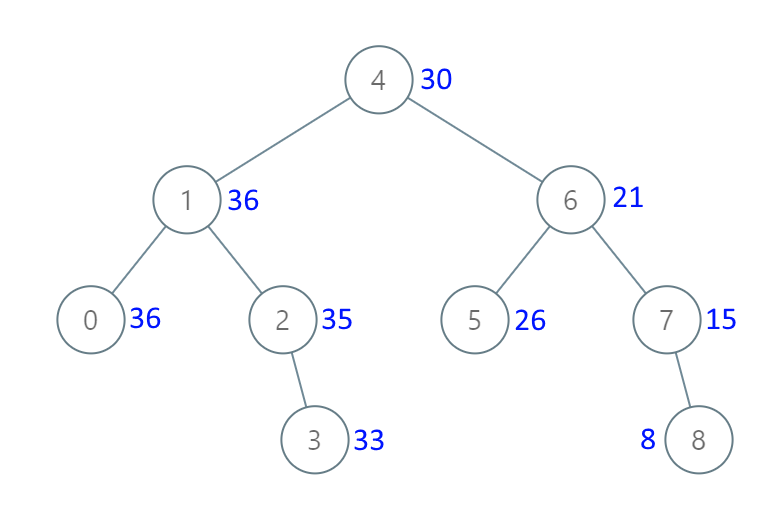# 538. Convert BST to Greater Tree

Convert BST to Greater Tree - LeetCode

Given the root of a Binary Search Tree (BST), convert it to a Greater Tree such that every key of the original BST is changed to the original key plus the sum of all keys greater than the original key in BST. As a reminder, a binary search tree is a tree that satisfies these constraints:

``````The left subtree of a node contains only nodes with keys less than the node's key.
The right subtree of a node contains only nodes with keys greater than the node's key.
Both the left and right subtrees must also be binary search trees.
``````

Example 1:Input: root = [4,1,6,0,2,5,7,null,null,null,3,null,null,null,8] Output: [30,36,21,36,35,26,15,null,null,null,33,null,null,null,8] Example 2: Input: root = [0,null,1] Output: [1,null,1]

Constraints:

``````The number of nodes in the tree is in the range [0, 104].
-104 <= Node.val <= 104
All the values in the tree are unique.
root is guaranteed to be a valid binary search tree.
``````

• code
``````class Solution {
int total = 0;
public TreeNode convertBST(TreeNode root) {
if (root != null){
convertBST(root.right);
total += root.val;
root.val = total;
convertBST(root.left);
}
return root;
}
}
``````
• code
``````class Solution(object):
def convertBST(self, root):
def change(node, val=0):
if node:
node.val += change(node.right, val)
return change(node.left, node.val)
else:
return val

change(root)
return root
``````
• code
``````class Solution(object):
def __init__(self):
self.total = 0

def convertBST(self, root):
if root is not None:
self.convertBST(root.right)
self.total += root.val
root.val = self.total
self.convertBST(root.left)
return root
``````